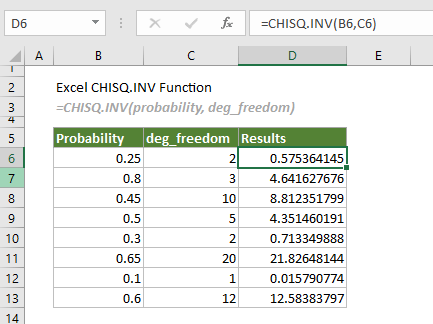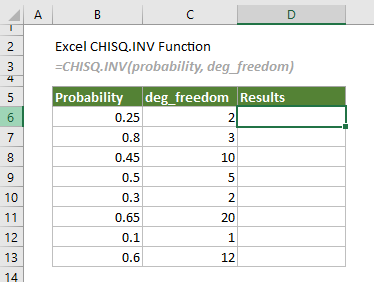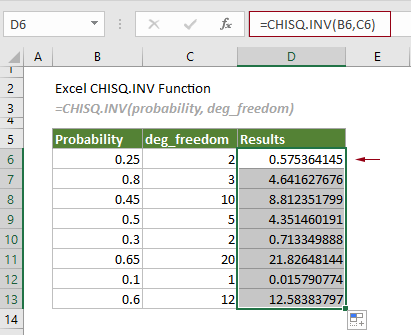## Excel CHISQ.INV function

The CHISQ.INV function calculates the inverse of the left-tailed probability of the chi-squared distribution.Note: This CHISQ.INV function is only available in Excel 2010 and later versions.

#### Syntax

=CHISQ.INV(probability, deg_freedom)

#### Arguments

Probability (required): A numeric value representing the probability associated with the chi-squared distribution.
-- The value supplied for the Probability argument must be > 0 and < 1.
De_freedom (required): The number of degrees of freedom.
-- The value supplied for this argument must be ≥ 1 and < 1010.

#### Remarks

1. If deg_freedom is not an integer, it will be truncated;
2. The #VALUE! error occurs if one of the supplied arguments is non-numeric.
3. The #NUM! error occurs when either of the following conditions is met:
-- Probability < 0 or ≥ 1;
-- Deg_freedom < 1 or > 1010.

#### Return value

It returns a numeric value.

#### Example

To calculate the inverse of the left-tailed probability of the chi-squared distribution based on the following given probabilities and degrees of freedom, you can do as follows.Select a cell, for example D6, enter the formula below and press the Enter key to get the first result. Select this result cell and then drag its AutoFill Handle down to get the rest of the results.

=CHISQ.INV(B6,C6)Note: Arguments in the formula above are supplied as cell references. You can directly type numbers as arguments in the formula. Here the formula in D6 can be changed to:

=CHISQ.INV(0.25,2)

#### Related Functions

Excel CHISQ.INV.RT function
The CHISQ.INV.RT function calculates the inverse of the right-tailed probability of the chi-squared distribution.

Excel CHISQ.TEST function
The CHISQ.TEST function calculates the chi-squared distribution of two provided data sets (the observed and expected frequencies.

Excel CONFIDENCE.NORM function
The CONFIDENCE.NORM function uses a normal distribution to calculate the confidence interval for a population mean.

Excel CONFIDENCE.T function
The CONFIDENCE.T function uses a student’s distribution to calculate the confidence interval for a population mean.

### The Best Office Productivity Tools

#### Kutools for Excel - Helps You To Stand Out From Crowd

 Popular Features: Find, Highlight or Identify Duplicates  |  Delete Blank Rows  |  Combine Columns or Cells without Losing Data  |  Round without Formula ... Super VLookup: Multiple Criteria  |  Multiple Value  |  Across Multi-Sheets  |  Fuzzy Lookup... Adv. Drop-down List: Easy Drop Down List  |  Dependent Drop Down List  |  Multi-select Drop Down List... Column Manager: Add a Specific Number of Columns  |  Move Columns  |  Toggle Visibility Status of Hidden Columns  |  Compare Columns to Select Same & Different Cells ... Featured Features: Grid Focus  |  Design View  |  Big Formula Bar  |  Workbook & Sheet Manager | Resource Library (Auto Text)  |  Date Picker  |  Combine Worksheets  |  Encrypt/Decrypt Cells  |  Send Emails by List  |  Super Filter  |  Special Filter (filter bold/italic/strikethrough...) ... Top 15 Toolsets:  12 Text Tools (Add Text, Remove Characters ...)  |  50+ Chart Types (Gantt Chart ...)  |  40+ Practical Formulas (Calculate age based on birthday ...)  |  19 Insertion Tools (Insert QR Code, Insert Picture from Path ...)  |  12 Conversion Tools (Numbers to Words, Currency Conversion ...)  |  7 Merge & Split Tools (Advanced Combine Rows, Split Excel Cells ...)  |  ... and more

Kutools for Excel Boasts Over 300 Features, Ensuring That What You Need is Just A Click Away...#### Office Tab - Enable Tabbed Reading and Editing in Microsoft Office (include Excel)

• One second to switch between dozens of open documents!
• Reduce hundreds of mouse clicks for you every day, say goodbye to mouse hand.
• Increases your productivity by 50% when viewing and editing multiple documents.
• Brings Efficient Tabs to Office (include Excel), Just Like Chrome, Edge and Firefox.